# Python lxml

Filed Under: Python

Python lxml is the most feature-rich and easy-to-use library for processing XML and HTML data. Python scripts are written to perform many tasks like Web scraping and parsing XML. In this lesson, we will study about python lxml library and how we can use it to parse XML data and perform web scraping as well.

## Python lxml libraryPython lxml is an easy to use and feature rich library to process and parse XML and HTML documents. lxml is really nice API as it provides literally everything to process these 2 types of data. The two main points which make lxml stand out are:

• Ease of use: It has very easy syntax than any other library present
• Performance: Processing even large XML files takes very less time

### Python lxml install

We can start using lxml by installing it as a python package using pip tool:

``````
pip install lxml
``````

Once we are done with installing this tool, we can get started with simple examples.

### Creating HTML Elements

With lxml, we can create HTML elements as well. The elements can also be calles as the Nodes. Let’s create basic structure of an HTML page using just the library:

``````
from lxml import etree

root_elem = etree.Element('html')
etree.SubElement(root_elem, 'title')
etree.SubElement(root_elem, 'body')
print(etree.tostring(root_elem, pretty_print=True).decode("utf-8"))
``````

When we run this script, we can see the HTML elements being formed:We can see HTML elements or nodes being made. The `pretty_print` parameter helps to print indented version of HTML document.

These HTML elements are basically a list. We can access this list normally:

``````
html = root_elem
print(html.tag)
``````

And this will just print `head` as that is the tag present right inside html tag. We can also print all elements inside the root tag:

``````
for element in root_elem:
print(element.tag)
``````

This will print all tags:### Checking validity of HTML Elements

With `iselement()` function, we can even check if given element is a valid HTML element:

``````
print(etree.iselement(root_elem))
``````

We just used the last script we wrote. This will give a simple output: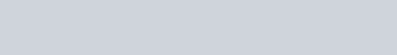### Using attributes with HTML Elements

We can add metadata to each HTML element we construct by adding attributes to the elements we make:

``````
from lxml import etree

html_elem = etree.Element("html", lang="en_GB")
print(etree.tostring(html_elem))
``````

When we run this, we see: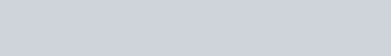We can now access these attributes as:

``````
print(html_elem.get("lang"))
``````

Value is printed to the console: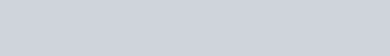Note that is the attribute doesn’t exist for given HTML element, we will get `None` as output.

We can also set attributes for an HTML element as:

``````
html_elem.set("best", "JournalDev")
print(html_elem.get("best"))
``````

When we print the value, we get the expected results: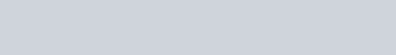### Sub-Elements with values

Sub-elements we constructed above were empty and that is no fun! Let’s make some sub-elements and put some values in it using lxml library.

``````
from lxml import etree

html = etree.Element("html")
etree.SubElement(html, "title").text = "I am the title!"
etree.SubElement(html, "body").text = "Here is the body"

print(etree.tostring(html, pretty_print=True).decode('utf-8'))
``````

This looks like some healthy data. Let’s see the output:### Feeding RAW XML for Serialisation

We can provide RAW XML data directly to etree and parse it as well as it completely understands what is passed to it.

``````
from lxml import etree

print(etree.tostring(html, pretty_print=True).decode('utf-8'))
``````

Let’s see the output:If you want the data to include the root XML tag declaration, even that is possible:

``````
from lxml import etree

print(etree.tostring(html, xml_declaration=True).decode('utf-8'))
``````

Let’s see the output now:### Python lxml etree parse() function

The `parse()` function can be used to parse from files and file-like objects:

``````
from lxml import etree
from io import StringIO

title = StringIO("<title>Title Here</title>")
tree = etree.parse(title)

print(etree.tostring(tree))
``````

Let’s see the output now: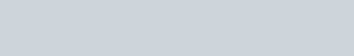### Python lxml etree fromstring() function

The `fromstring()` function can be used to parse Strings:

``````
from lxml import etree

title = "<title>Title Here</title>"
root = etree.fromstring(title)
print(root.tag)
``````

Let’s see the output now: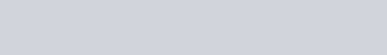### Python lxml etree XML() function

The `fromstring()` function can be used to write XML literals directly into the source:

``````
from lxml import etree

title = etree.XML("<title>Title Here</title>")
print(title.tag)
print(etree.tostring(title))
``````

Let’s see the output now:Reference: LXML Documentation.

1.venkat says: